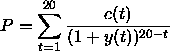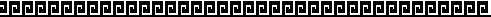Pricing of a bond

This Excel-Sheet calculates the fair price of a bond over time. As input parameters it uses the cashflows at different timesteps and a yield curve. Actually the sheet calculates the price of one bond according to two different yieldcurves. You can specify a spread (curve) between the two yieldcurves.
If we name the value of the yieldcurve at time t with y(t), the cashflow at time t with c(t), t=0..20, then the price of a bond with cashflows c(t) is given by:[ Startpage ] --- [ gästebuch ] --- [ sitemap ] --- up to date: 04-08-2003